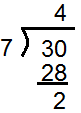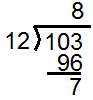# Tutoring math, you know that div and mod are also fundamental to computer science.  The tutor presents them with an example.

Consider the age-old long division situation:In this case, the question posed is “30 divided by 7”. The answer is “4, remainder 2.” But in terms of div and mod, what does it mean?

4 is the answer to 30 div 7. The div operation gives the integer quotient of a division: it gives the number of times the divisor can go in. The mod operator, on the other hand, gives the remainder of a division: 30 mod 7 is 2, just as shown above.

A really neat example of div and mod in action is the following:

Example: Convert 103 inches to feet and inches

Solution: Set it up long division style, like so:The answer is that 103 inches is 8 feet, 7 inches. 103 div 12=8 gives the feet, while 103 mod 12=7 gives the inches. Once again: div gives the integer quotient, whereas mod gives the remainder.

For more implications of div and mod, come visit again:)

Jack of Oracle Tutoring by Jack and Diane, Campbell River, BC.